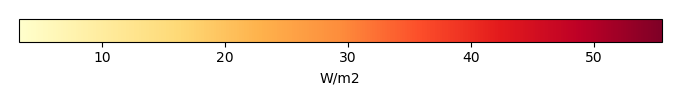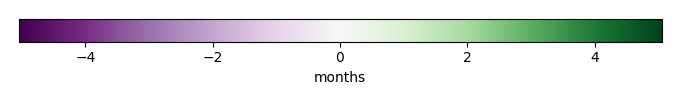# Mean State

Period Mean (original grids) [W/m2]
Model Period Mean (intersection) [W/m2]
Model Period Mean (complement) [W/m2]
Benchmark Period Mean (intersection) [W/m2]
Benchmark Period Mean (complement) [W/m2]
Bias [W/m2]
RMSE [W/m2]
Phase Shift [months]
Bias Score 
RMSE Score 
Seasonal Cycle Score 
Spatial Distribution Score 
Overall Score 
Benchmark [-] 70.6
CLM5PHSOFF [-] 71.0 71.7 63.6 70.7 18.1 0.913 19.1 1.22 0.31 0.12 0.84 0.99 0.47
CLM5PHSON [-] 70.8 71.3 62.2 70.7 18.1 -0.0585 16.6 1.34 0.38 0.17 0.81 0.99 0.50
Period Mean (original grids) [W/m2]
Model Period Mean (intersection) [W/m2]
Model Period Mean (complement) [W/m2]
Benchmark Period Mean (intersection) [W/m2]
Benchmark Period Mean (complement) [W/m2]
Bias [W/m2]
RMSE [W/m2]
Phase Shift [months]
Bias Score 
RMSE Score 
Seasonal Cycle Score 
Spatial Distribution Score 
Overall Score 
Benchmark [-] 47.3
CLM5PHSOFF [-] 46.3 45.9 29.8 47.3 34.2 -1.52 22.4 1.36 0.72 0.47 0.74 0.92 0.66
CLM5PHSON [-] 46.7 46.1 37.1 47.3 34.2 -1.40 22.7 1.43 0.70 0.45 0.73 0.92 0.65
Period Mean (original grids) [W/m2]
Model Period Mean (intersection) [W/m2]
Model Period Mean (complement) [W/m2]
Benchmark Period Mean (intersection) [W/m2]
Benchmark Period Mean (complement) [W/m2]
Bias [W/m2]
RMSE [W/m2]
Phase Shift [months]
Bias Score 
RMSE Score 
Seasonal Cycle Score 
Spatial Distribution Score 
Overall Score 
Benchmark [-] 52.1
CLM5PHSOFF [-] 47.2 46.6 50.5 52.2 23.6 -5.73 21.8 1.05 0.57 0.44 0.86 0.96 0.65
CLM5PHSON [-] 48.3 48.2 51.1 52.2 23.6 -4.01 21.5 1.24 0.58 0.45 0.82 0.97 0.65
Period Mean (original grids) [W/m2]
Model Period Mean (intersection) [W/m2]
Model Period Mean (complement) [W/m2]
Benchmark Period Mean (intersection) [W/m2]
Benchmark Period Mean (complement) [W/m2]
Bias [W/m2]
RMSE [W/m2]
Phase Shift [months]
Bias Score 
RMSE Score 
Seasonal Cycle Score 
Spatial Distribution Score 
Overall Score 
Benchmark [-] 14.9
CLM5PHSOFF [-] 3.70 12.4 9.61 15.0 3.59 -2.61 10.2 0.215 0.81 0.69 1.0 0.53 0.74
CLM5PHSON [-] 15.5 15.7 11.3 15.0 3.59 0.460 12.0 0.219 0.86 0.61 1.0 0.70 0.76
Period Mean (original grids) [W/m2]
Model Period Mean (intersection) [W/m2]
Model Period Mean (complement) [W/m2]
Benchmark Period Mean (intersection) [W/m2]
Benchmark Period Mean (complement) [W/m2]
Bias [W/m2]
RMSE [W/m2]
Phase Shift [months]
Bias Score 
RMSE Score 
Seasonal Cycle Score 
Spatial Distribution Score 
Overall Score 
Benchmark [-] 77.5
CLM5PHSOFF [-] 104. 103. 107. 80.4 34.5 22.7 30.3 2.12 0.21 0.13 0.67 0.41 0.31
CLM5PHSON [-] 95.5 94.9 98.6 80.4 34.5 15.1 25.4 2.16 0.26 0.17 0.66 0.28 0.31
Period Mean (original grids) [W/m2]
Model Period Mean (intersection) [W/m2]
Model Period Mean (complement) [W/m2]
Benchmark Period Mean (intersection) [W/m2]
Benchmark Period Mean (complement) [W/m2]
Bias [W/m2]
RMSE [W/m2]
Phase Shift [months]
Bias Score 
RMSE Score 
Seasonal Cycle Score 
Spatial Distribution Score 
Overall Score 
Benchmark [-] 26.3
CLM5PHSOFF [-] 20.8 20.8 22.8 26.3 3.49 -5.56 10.5 0.122 0.80 0.76 0.99 0.94 0.85
CLM5PHSON [-] 25.1 25.0 25.4 26.3 3.49 -1.27 10.8 0.351 0.88 0.71 0.98 0.99 0.85
Period Mean (original grids) [W/m2]
Model Period Mean (intersection) [W/m2]
Model Period Mean (complement) [W/m2]
Benchmark Period Mean (intersection) [W/m2]
Benchmark Period Mean (complement) [W/m2]
Bias [W/m2]
RMSE [W/m2]
Phase Shift [months]
Bias Score 
RMSE Score 
Seasonal Cycle Score 
Spatial Distribution Score 
Overall Score 
Benchmark [-] 28.0
CLM5PHSOFF [-] 24.6 24.0 32.8 28.1 13.0 -4.18 13.7 0.572 0.64 0.65 0.96 0.87 0.75
CLM5PHSON [-] 26.2 26.5 20.0 28.1 13.0 -1.20 14.1 0.647 0.71 0.61 0.96 0.93 0.76
Period Mean (original grids) [W/m2]
Model Period Mean (intersection) [W/m2]
Model Period Mean (complement) [W/m2]
Benchmark Period Mean (intersection) [W/m2]
Benchmark Period Mean (complement) [W/m2]
Bias [W/m2]
RMSE [W/m2]
Phase Shift [months]
Bias Score 
RMSE Score 
Seasonal Cycle Score 
Spatial Distribution Score 
Overall Score 
Benchmark [-] 57.0
CLM5PHSOFF [-] 56.8 53.8 76.1 57.2 29.3 -3.65 24.8 1.33 0.49 0.40 0.82 0.95 0.61
CLM5PHSON [-] 56.9 56.1 71.3 57.2 29.3 0.854 25.7 1.75 0.57 0.36 0.73 0.96 0.59
Period Mean (original grids) [W/m2]
Model Period Mean (intersection) [W/m2]
Model Period Mean (complement) [W/m2]
Benchmark Period Mean (intersection) [W/m2]
Benchmark Period Mean (complement) [W/m2]
Bias [W/m2]
RMSE [W/m2]
Phase Shift [months]
Bias Score 
RMSE Score 
Seasonal Cycle Score 
Spatial Distribution Score 
Overall Score 
Benchmark [-] 15.7
CLM5PHSOFF [-] 13.9 14.5 8.14 15.8 -6.07 -1.26 11.9 0.151 0.88 0.63 1.0 0.68 0.76
CLM5PHSON [-] 16.7 17.1 9.17 15.8 -6.07 1.09 14.3 0.101 0.92 0.55 1.0 0.75 0.76
Period Mean (original grids) [W/m2]
Model Period Mean (intersection) [W/m2]
Model Period Mean (complement) [W/m2]
Benchmark Period Mean (intersection) [W/m2]
Benchmark Period Mean (complement) [W/m2]
Bias [W/m2]
RMSE [W/m2]
Phase Shift [months]
Bias Score 
RMSE Score 
Seasonal Cycle Score 
Spatial Distribution Score 
Overall Score 
Benchmark [-] 26.8
CLM5PHSOFF [-] 33.5 31.4 49.1 26.9 19.3 4.58 18.7 0.687 0.66 0.37 0.93 0.94 0.66
CLM5PHSON [-] 32.6 31.6 52.1 26.9 19.3 6.35 16.8 0.693 0.67 0.41 0.94 0.92 0.67
Period Mean (original grids) [W/m2]
Model Period Mean (intersection) [W/m2]
Model Period Mean (complement) [W/m2]
Benchmark Period Mean (intersection) [W/m2]
Benchmark Period Mean (complement) [W/m2]
Bias [W/m2]
RMSE [W/m2]
Phase Shift [months]
Bias Score 
RMSE Score 
Seasonal Cycle Score 
Spatial Distribution Score 
Overall Score 
Benchmark [-] 31.2
CLM5PHSOFF [-] 27.3 27.3 27.9 31.7 9.65 -4.50 14.4 0.416 0.69 0.68 0.97 0.58 0.72
CLM5PHSON [-] 33.4 33.4 33.3 31.7 9.65 1.79 15.3 0.325 0.79 0.61 0.98 0.72 0.74
Period Mean (original grids) [W/m2]
Model Period Mean (intersection) [W/m2]
Model Period Mean (complement) [W/m2]
Benchmark Period Mean (intersection) [W/m2]
Benchmark Period Mean (complement) [W/m2]
Bias [W/m2]
RMSE [W/m2]
Phase Shift [months]
Bias Score 
RMSE Score 
Seasonal Cycle Score 
Spatial Distribution Score 
Overall Score 
Benchmark [-] 39.1
CLM5PHSOFF [-] 36.7 38.4 17.6 39.4 16.4 -1.07 16.5 0.811 0.57 0.42 0.87 0.97 0.65
CLM5PHSON [-] 33.9 40.2 7.50 39.4 16.4 1.20 16.5 0.895 0.61 0.42 0.85 0.97 0.66
Period Mean (original grids) [W/m2]
Model Period Mean (intersection) [W/m2]
Model Period Mean (complement) [W/m2]
Benchmark Period Mean (intersection) [W/m2]
Benchmark Period Mean (complement) [W/m2]
Bias [W/m2]
RMSE [W/m2]
Phase Shift [months]
Bias Score 
RMSE Score 
Seasonal Cycle Score 
Spatial Distribution Score 
Overall Score 
Benchmark [-] 8.45
CLM5PHSOFF [-] 7.82 12.3 3.87 8.47 5.81 3.76 10.2 1.15 0.58 0.42 0.92 0.85 0.64
CLM5PHSON [-] 8.46 14.3 3.21 8.47 5.81 6.13 13.3 1.12 0.55 0.26 0.93 0.93 0.58
Period Mean (original grids) [W/m2]
Model Period Mean (intersection) [W/m2]
Model Period Mean (complement) [W/m2]
Benchmark Period Mean (intersection) [W/m2]
Benchmark Period Mean (complement) [W/m2]
Bias [W/m2]
RMSE [W/m2]
Phase Shift [months]
Bias Score 
RMSE Score 
Seasonal Cycle Score 
Spatial Distribution Score 
Overall Score 
Benchmark [-] 14.0
CLM5PHSOFF [-] 13.9 14.7 8.10 14.0 1.27 0.581 11.3 0.239 0.83 0.61 0.99 0.82 0.77
CLM5PHSON [-] 15.9 16.3 7.53 14.0 1.27 1.78 12.9 0.293 0.85 0.54 0.99 0.89 0.76
Period Mean (original grids) [W/m2]
Model Period Mean (intersection) [W/m2]
Model Period Mean (complement) [W/m2]
Benchmark Period Mean (intersection) [W/m2]
Benchmark Period Mean (complement) [W/m2]
Bias [W/m2]
RMSE [W/m2]
Phase Shift [months]
Bias Score 
RMSE Score 
Seasonal Cycle Score 
Spatial Distribution Score 
Overall Score 
Benchmark [-] 50.4
CLM5PHSOFF [-] 60.3 57.9 69.6 50.9 20.2 6.66 23.3 1.01 0.47 0.32 0.87 0.95 0.58
CLM5PHSON [-] 57.0 56.5 63.8 50.9 20.2 8.13 21.5 0.909 0.50 0.36 0.89 0.96 0.61
Period Mean (original grids) [W/m2]
Model Period Mean (intersection) [W/m2]
Model Period Mean (complement) [W/m2]
Benchmark Period Mean (intersection) [W/m2]
Benchmark Period Mean (complement) [W/m2]
Bias [W/m2]
RMSE [W/m2]
Phase Shift [months]
Bias Score 
RMSE Score 
Seasonal Cycle Score 
Spatial Distribution Score 
Overall Score 
Benchmark [-] 49.7
CLM5PHSOFF [-] 45.6 44.5 60.1 49.8 19.0 -5.34 17.0 0.330 0.74 0.70 0.98 0.92 0.81
CLM5PHSON [-] 50.0 49.8 59.9 49.8 19.0 0.529 15.5 0.470 0.83 0.70 0.96 0.85 0.81
Period Mean (original grids) [W/m2]
Model Period Mean (intersection) [W/m2]
Model Period Mean (complement) [W/m2]
Benchmark Period Mean (intersection) [W/m2]
Benchmark Period Mean (complement) [W/m2]
Bias [W/m2]
RMSE [W/m2]
Phase Shift [months]
Bias Score 
RMSE Score 
Seasonal Cycle Score 
Spatial Distribution Score 
Overall Score 
Benchmark [-] 25.2
CLM5PHSOFF [-] 25.5 25.4 30.4 25.4 10.7 -0.0146 11.2 0.145 0.80 0.70 0.99 0.93 0.83
CLM5PHSON [-] 30.4 30.4 34.3 25.4 10.7 4.98 13.7 0.267 0.81 0.62 0.98 0.92 0.79
Period Mean (original grids) [W/m2]
Model Period Mean (intersection) [W/m2]
Model Period Mean (complement) [W/m2]
Benchmark Period Mean (intersection) [W/m2]
Benchmark Period Mean (complement) [W/m2]
Bias [W/m2]
RMSE [W/m2]
Phase Shift [months]
Bias Score 
RMSE Score 
Seasonal Cycle Score 
Spatial Distribution Score 
Overall Score 
Benchmark [-] 27.2
CLM5PHSOFF [-] 22.9 23.3 31.4 27.2 14.3 -3.83 12.5 0.648 0.68 0.59 0.95 0.95 0.75
CLM5PHSON [-] 25.9 26.4 31.7 27.2 14.3 -0.645 13.1 0.668 0.73 0.54 0.95 0.98 0.75
Period Mean (original grids) [W/m2]
Model Period Mean (intersection) [W/m2]
Model Period Mean (complement) [W/m2]
Benchmark Period Mean (intersection) [W/m2]
Benchmark Period Mean (complement) [W/m2]
Bias [W/m2]
RMSE [W/m2]
Phase Shift [months]
Bias Score 
RMSE Score 
Seasonal Cycle Score 
Spatial Distribution Score 
Overall Score 
Benchmark [-] 90.0
CLM5PHSOFF [-] 93.2 93.3 93.4 90.2 27.7 3.06 18.7 1.80 0.41 0.13 0.74 0.99 0.48
CLM5PHSON [-] 91.4 91.5 87.6 90.2 27.7 1.06 14.5 1.90 0.44 0.20 0.73 0.92 0.50
Period Mean (original grids) [W/m2]
Model Period Mean (intersection) [W/m2]
Model Period Mean (complement) [W/m2]
Benchmark Period Mean (intersection) [W/m2]
Benchmark Period Mean (complement) [W/m2]
Bias [W/m2]
RMSE [W/m2]
Phase Shift [months]
Bias Score 
RMSE Score 
Seasonal Cycle Score 
Spatial Distribution Score 
Overall Score 
Benchmark [-] 69.7
CLM5PHSOFF [-] 62.3 61.7 66.9 69.8 17.5 -7.97 22.6 0.967 0.59 0.44 0.90 0.99 0.67
CLM5PHSON [-] 65.2 64.8 69.8 69.8 17.5 -4.76 20.5 0.946 0.64 0.47 0.90 0.99 0.69
Period Mean (original grids) [W/m2]
Model Period Mean (intersection) [W/m2]
Model Period Mean (complement) [W/m2]
Benchmark Period Mean (intersection) [W/m2]
Benchmark Period Mean (complement) [W/m2]
Bias [W/m2]
RMSE [W/m2]
Phase Shift [months]
Bias Score 
RMSE Score 
Seasonal Cycle Score 
Spatial Distribution Score 
Overall Score 
Benchmark [-] 22.7
CLM5PHSOFF [-] 23.3 24.4 14.3 23.0 8.02 1.50 12.7 0.870 0.51 0.46 0.91 0.95 0.66
CLM5PHSON [-] 27.6 27.9 17.3 23.0 8.02 4.79 15.2 0.929 0.51 0.38 0.92 0.95 0.63

# Temporally integrated period mean

BENCHMARK MEANMODEL MEANBIASBIAS SCORERMSERMSE SCOREBENCHMARK MAX MONTHMODEL MAX MONTHDIFFERENCE IN MAX MONTHSEASONAL CYCLE SCORESPATIAL TAYLOR DIAGRAMMODEL COLORS# Spatially integrated regional mean

MODEL COLORSREGIONAL MEANANNUAL CYCLEMONTHLY ANOMALYANNUAL CYCLE# All Models

BenchmarkCLM5PHSOFFCLM5PHSON# Data Information

creation_date: Thu Dec 5 00:02:00 PST 2013

source_file: This product is generated from monthly FluxNet MTE observations

title: derived FluxNet MTE in 0.5x0.5

Approach: I read this variable from data file, and then save the data in NetCDF format by each month and each year. Meanwhile, I also converted the unit from g C m-2 day-1 to Kg C m-2 s-1.

Temporal resolution: monthly

General information: This product was derived from Fluxnet-MTE originally from Monthly estimates of global biosphere-atmosphere fluxes from Biogeochemistry group at Max Planck Institute in Jena, Germany.

Spatial resolution: 0.5x0.5 degree